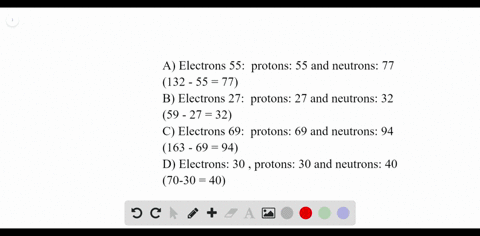### How many electrons, protons, and neutrons are co…

00:39University of Miami
Problem 72

Fill in the blanks in Table 4.6.

## Discussion

You must be signed in to discuss.

## Video Transcript

so no work on problems. 72 from Chapter four Ms Problem. We're asked to fill in a table with some information for some isotopes of chlorine and zirconium. So first we can, drawing what we're given in this table and then feeling what we don't know. So we have to isotopes of chlorine and two for zirconium, and we want to fill in things like atomic number, mass number, pro tones, neutrons and electrons. So first we can fill in the values which we are already given. So we have. In the first column, we have 17 and 35 for atomic number and for mass number. Then we have 37 17 for mass number and electrons. In the second column for the third column for a zirconium, we have 40 for atomic number 50 for New Schranz. And for the last column, we have 92 for mass number and 44 prove Tom's so we can start with the first column. If our atomic number 17 the number of protons must equal 17 as well as the number of electrons, the number of neutrons must equal the mass number minus the atomic number, so that is equal to 18. Atomic number for chlorine will remain the same. So it's 17 as is the number of protons here. What changes is the number of neutrons because we have a different mass number, so we subtract atomic number for mass. Number 37 minus 17 gives us 20 neutrons in the third column or given neutrons and atomic number. If we add those together, we can get the mass number for this isotope of zirconium. Number of protons should be able to the atomic number, and the number of electrons should be equal to the number of protons, which is 40. The atomic number of zirconium does not change, so it's 40. In the last comb number of neutrons. We confined by subtracting a mass number minus atomic number. So 92 minus 40 equals 50 to 2 more neutrons than our third column, and the number of electrons remains unchanged. So this is the